## Indicators

•Cited by SciELO
•Access statistics

## Related links

•Similars in SciELO

## Print version ISSN 0035-001X

### Rev. mex. fis. vol.63 n.3 México May./Jun. 2017

Research

Analyses of 𝛑 ± nucleus elastic scattering data at 𝐓 𝛑 =𝟓𝟎 MeV using a suggested scaling method

1Physics Department, Taif University, Taif, Zip Code 21974, Saudi Arabia e-mail: zfs07@hotmail.com

Abstract

The data for elastically scattered charged pions from few nuclei, namely 16 O, 28 Si, 30 Si, 32 S, 34 S, 40 Ca, 43 Ca, 56 Fe, 58 Ni, 64 Ni and 90 Zr have been analyzed by obtained potentials using a suggested scaling procedure. Originally the π ± - 12 C elastic scattering data at 50 MeV was nicely fitted by a parameterized simple local optical potential extracted from available phase shifts using inverse scattering theory. The potential parameters of the π ± - 12 C systems were scaled to π ± - 16 O systems and then successively to other few systems covering the scattering of charged pions from target nuclei and isotopes, namely π ± - 28 Si, 30 Si, π ± - 32 S, 34 S, π ± - 40 Ca, 48 Ca, π ± - 56 Fe, π ± - 58 Ni, 64 Ni and π ± - 90 Zr. For all these systems, the obtained scaled potentials showed a remarkable success in explaining the available elastic scattering data at 50 MeV. For the first time, simple scaling relations are well established, and are used in explaining successfully the elastic differential and integral cross sections. This motivates using the scaling procedure to predict pion-nucleus potentials capable of explaining measured angular distributions for the scattering of charged pions off other target nuclei, and few isotopes, at energies in the low energy region.

Keywords: Pion-nucleus potential; Klein-Gordon equation; elastic scattering; inverse scattering theory; phase shift analysis; scaling; method; low-energy physics

1. Introduction

In the last few decades, a great attention has been given to pion physics, mainly in using the pion as a probe to glean important nuclear information1. This is due to its intrinsic properties2 as spin, charge and mass, in addition to other gained properties as kinetic energy which is provided by the pions’ producing facilities. These properties have given it superiority over other probes, as electrons and protons, to investigate the interior of the nucleus as well as the peripheral areas. As such, pion factories and associated facilities3 have been used in providing a great deal of accurate pion-nucleus scattering data. In fact these data are considered correct points, and the success of any theoretical model is measured by its capability in explaining these data points.

It is well-known that the pion’s mean free path, λ, changes non-monotonically with its incident kinetic energy, 𝑇 𝜋 . Obviously in the delta resonance energy region ( 𝑇 𝜋 =200±100 MeV), 𝜆 is less than the inter-nucleon distance 𝑑 and, as such, the incident pion faces a complete absorption at the surface, i.e. the nucleus surface acts as a complete black disc4. On the contrary, and in the low energy region, ( 𝑇 𝜋 <100 MeV), 𝜆 is of few Fermis and, as such, the pion penetrates deeply inside the nucleus, i.e. the nucleus is transparent to pions5. Such a merit gave the pion a better prospect to reveal nuclear mysteries and solve subtler effects.

Coping with a wealth of pion-nucleus scattering data, provided by available pion facilities, many theories and theoretical models6 have been proposed to explain these data. It is well known that Klein-Gordon equation is the appropriate one to describe the relativistic scattering process of spinless particles, as pions, from target nuclei. The solution of this equation is solely based on the determination of the pion-nucleus potential which has an explicit appearance in the equation. Hence, many theoretical approaches had competed to provide the most adequate potential which provides a better explanation for available pion-nucleus data. The most recent of these potentials is Satchler’s7 simple local potential of Woods-Saxon form for both real and imaginary parts. The limitations of Satchler’s potential have been rectified by using a new simple local potential8 guided by inverted potential points obtained from available phase shifts using inverse scattering theory within the framework of the full Klein-Gordon equation.

Unfortunately, the inverse scattering theory is of no use if phase shifts are absent. As such, this obligated the need for an alternative method based on benefitting from cases with available phase shifts for ones without available phase shift analyses. For several non-relativistic nucleon-nucleus, alpha-nucleus and nucleus-nucleus systems, a scaling method11-15 was used to obtain the potential parameters for one system from the potential parameters, used successfully in explaining the measured angular distributions, of a nearby system. Guided by this, we will scale the potential parameters for a pion-nucleus system, at 50 MeV, from another nearby successful one. The extent of success for this method is measured by the capability of the scaled potential to account for the experimental angular distributions. To test the method, we have scaled the 𝜋 ± - 16 O potential parameters from 𝜋 ± - 12 C potential parameters16,17. The obtained potentials by scaling are compared to the ones from inverse scattering theory. Both are in a good agreement, and they fit the measured angular distributions reasonably well. With this reasonable agreement, the use of the scaling method is then continued successively to cover the scattering of charged pions, with 50 MeV incident kinetic energy, from other target nuclei as 28 Si, 32 S, 40 Ca, 56 Fe, 58 Ni, 90 Zr and few isotopes as 30 Si, 34 S, 48 Ca and 64 Ni. Our results are encouraging, and they strongly support the generalization of the method to be used in studying low-energy pion-nucleus scattering cases.

In the following section, theory is outlined. In its subsequent section, light is shed on results and discussion. The last section contains the concluding remarks.

2. Theory

The theory of this work is very much similar, especially the relations, to that of our recent published paper17. This is due to the similarity of the topics. Nevertheless, it is again outlined here for emphasis and completeness.

As usual, the potential, 𝑉(𝑟), which has been adopted in analyzing successfully 𝜋 ± − 12 C elastic scattering data16,17 consists of a nuclear term, 𝑉 𝑁 (𝑟), in addition to the Coulomb one, 𝑉 𝐶 (𝑟):

V(r)=VN(r)+VC(r)

The nuclear part is a sum of three well-known phenomenological terms, two real terms and one imaginary term, and has the following analytical form:

VN(r)=V01+exp(r-R0a0)+V191;1+exp(r-1a112+iW3exp(r-R3a3)1+exp(r-R3a3);2

The first term is an attractive Woods-Saxon (WS) while the second one is a repulsive Squared Woods-Saxon (SWS), and the third is the surface Woods-Saxon. The Coulomb term, 𝑉 𝐶 (𝑟), is considered due to a uniformly insulating charged sphere. For the scattering of an incident charged pion from a stationary target nucleus of atomic number 𝑍 𝑇 , 𝑉 𝐶 (𝑟) has the following form:

VC(r)=*20l±ZTe28πε0RC3-r2RC2rRC±ZTe24πε0rr>RC

The Coulomb radius, 𝑅 𝐶 , is numerically estimated by 𝑅 𝐶 =1.2 𝐴 1/3 where 𝐴 is the atomic mass of the target nucleus in atomic mass units. The constants 𝑒 2 and 𝜀 0 are the squared electron charge and permittivity of free space; and 𝑒 2 /4𝜋 𝜀 0 is taken 1.44 MeV.fm in nuclear units. For the purpose of calculating scattering quantities as the scattering amplitude, the differential and reaction cross sections, 𝑉(𝑟) is implemented in the radial part of Klein-Gordon equation:

d2dr2+k2-U(r)-l(l+1)r2Rnl(r)=0

with 𝑘 2 and 𝑈(𝑟) are given by

k2=(E2-m2c4)/2c2U(r)=2E2c2V(r)-V2(r)/2E

The quantities 𝐸, 𝑚, ℏ=ℎ/2𝜋 and 𝑐 are the actual pion total energy, effective pion mass, reduced Planck constant which equals Planck’s constant ℎ(ℎ=6.626× 10 −34 J⋅ s) divided by 2𝜋 and the velocity of electromagnetic wave in vacuum (𝑐=3.0× 10 8 m/s), respectively. To avoid singularities and to put Eq. (4) in a more familiar convenient mathematical form and an easier computational format, 𝑅 𝑛ℓ (𝑟) is transformed into 𝜑 𝑛ℓ (𝑟) by the following substitution:

Rnl(r)=(kr)l+1φnl(r)

So Eq. (4) reads:

d2dr2+2(l+1)rddrk2-U(r)φnl(r)=0

To calculate the previously mentioned scattering quantities, one needs first to find the phase shift, 𝛿 ℓ , for each contributing partial wave ℓ. This is usually done by matching the inner and outer solutions of Eq. (8), namely the logarithmic derivatives, at the surface 𝑟=𝑅 which is also called the matching point or the cutoff radius. The outer solution, i.e. for 𝑟≥𝑅, is the well-known Coulomb wave function expressed as

φnl(r)=1(kr)l+1{Fl(η,kr)+exp(2iδl)-12i×Gl(η,kr)+iFl(η,kr)}

where 𝐹 ℓ and 𝐺 ℓ are the regular and irregular Coulomb wave functions, respectively. Although the effect of using the relativistic versions of these Coulomb wave functions compared to the non-relativistic ones is negligible, we have used the relativistic ones, as it should be, generated by the code when the nuclear part turns off18,19. The parameter 𝜂 is Sommerfeld parameter given by,

η=ZTαEk

where 𝛼 is the fine structure constant. On the other hand, the inner solution for Eq. (4) is obtained by integrating the equation numerically, from the origin 𝑟=0 to the cutoff radius 𝑟=𝑅, using Numerov’s method20.

Knowing 𝛿 ℓ , one can calculate the full elastic scattering amplitude, 𝑓(𝜃), at angle 𝜃, in the center of mass system, using the relation :

f(θ)=fc(θ)+12ikl=0(2l+1)e2iσγ×e2iδl-1Pl(cosθ)

where 𝑓 𝑐 (𝜃) is the pure Coulomb scattering amplitude, 𝑃 ℓ (cos𝜃) is the Legendre polynomial, and 𝜎 𝛾 is the Coulomb phase shift defined by21:

σγ=argΓγ+12+iη-12πγ-12-l

and the dimensionless parameter 𝛾 is given by:

γ=l+122-ZT2α2

It is clear that the second term under the square root is very small compared to the first term, except for ℓ=0. As such it can be neglected, and 𝛾 can be approximated as 𝛾≈(ℓ+(1/2)). With this logical approximation, the Coulomb scattering for the relativistic case, 𝜎 𝛾 , reduces to the Coulomb scattering for the nonrelativistic scattering case, 𝜎 ℓ 22.

The elastic differential cross section, 𝑑𝜎/𝑑𝛺, which equals the absolute square of the scattering amplitude, is expressed mathematically as :

dσdΩ=|f(θ)|2

Also the reaction cross sections, 𝜎 𝑟 , can be calculated using the well-known definition:

σr=πk2σl=0(2l+1)1-|Sl|2

where 𝑆 ℓ = 𝑒 2𝑖 𝛿 ℓ is the S-matrix, and 𝛿 ℓ is complex.

3. Results and Discussion

The scaling method pointed out by Haider and Malik11, which was only concerned with non-relativistic scattering problems, shows the dependence of the potential parameters, mainly the radii and the depths of the potential terms, on the geometry of the two colliding nuclei. Such a scaling method has been adopted in determining the potential parameters for a certain alpha-nucleus or nucleus-nucleus nuclear system from another nearby similar one. Following this strategy, the potentials for several two-nucleus systems had been determined by scaling the potential parameters of a successful nuclear nearby system. In fact, the potential parameters for 16 O - 16 O system9 have been scaled from 12 C - 12 C potential parameters. Also 𝛼− 32 S, 34 S potential parameters12 have been scaled from 𝛼- 28 Si potential parameters10. In 1995, Shehadeh8 has reemphasized the power and success of the scaling procedure in determining the correct potentials capable of providing nice fits to the experimental scattering data for 34 S- 64 Ni, 36 S - 58,64 Ni, 40 Ca- 48 Ca and 27 Al - 58 Ni nuclear systems13-15. Guided by the success of the scaling method for all the above mentioned non-relativistic scattering cases, described by the Schrödinger equation, we have applied it here to test its suitability and capability in explaining low-energy pion-nucleus elastic scattering data. This is based on obtaining the potential parameters for a certain pion-nucleus scattering case by scaling the potential parameters used successfully for another nearby similar system. Here we have first scaled the parameters of 𝜋 ± − 12 C potentials [16,17] to obtain the potential parameters of systems23. The obtained potential parameters of 𝜋 ± − 16 O sistems. Cases have determined the exact nature of the potential and have nicely explained the measured angular distributions. In addition, it is very interesting to see the good match between the scaled potential and the parameterized one based on the inverted potential points obtained from available phase shifts24,25 using inverse scattering theory26. Such a pleasant success motivates the use of the scaling method to determine approximately the nature of other pion-nucleus systems and, then, fit the data. This scaling method has been implemented with a start from 𝜋 ± − 12 C, and then carried out to cover the elastic scattering of charged pions from few nuclei, namely 16 O, 28 Si, 32 S, 40 Ca, 56 Fe, 58 Ni, except for 90 Zr nuclear target in a successive manner. The potential parameters along with calculated and measured reaction cross sections are listed in Table I; and the scaled potentials are shown in Fig. 1. Correspondingly the calculated and measured differential cross sections27-32 for both charged pions scattered elastically from all nuclei under consideration are depicted in Fig. 2, and the agreements are very reasonable. With no doubt, a remarkable improvement can easily be achieved by slight adjustment of one or two parameters, i.e. by increasing the number of free parameters. In Fig. 3 the scaled potentials, real and imaginary parts, are drawn along with the calculated differential cross sections, compared to the measured ones29-32, for the 50 MeV incident charged pions scattered off the four isotopes 30 Si, 34 S, 48 Ca and 64 Ni. Nevertheless, the agreement between the calculated and measured reaction cross sections is very obvious except for nuclear target where theory underestimates measured values for pions of both polarities. This is clearly depicted in Fig. 4. The potential parameters and the calculated reaction cross sections, including quasi-elastic cross sections32, compared to the measured ones where available33-38, are indicated in Tables I and II.

Hence, one can use the scaling method confidently to obtain the appropriate potentials that give a reasonable description for low-energy elastic-scattering data of other pion-nuclear systems.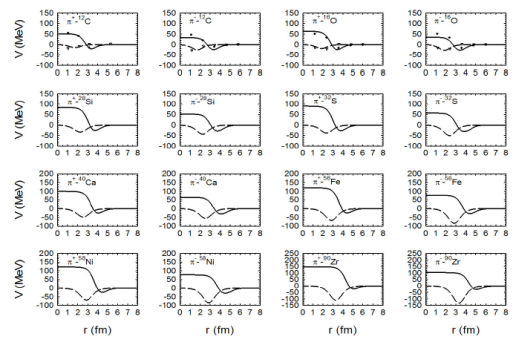Figure 1 The real and imaginary parts of the scaled potentials, drawn as solid and dashed lines, respectively, used in analyzing ¼§ ¡ 12C, 16O, 28Si, 32S, 40Ca, 56Fe, 58Ni, and 90Zr elastic scattering data at 50 MeV. The inverted real and imaginary potential points, obtained from available phase shifts [24,25], are represented by solid circles and empty triangles, respectively.Figure 2 The calculated differential cross sections, drawn as solid and dashed lines for positive and negative pions, respectively, compared to the experimental data, represented by solid circles and empty triangles [27-31,33], as a function of center of mass angle at 50 MeV incident charged pions scattered off 12C, 16O, 28Si, 32S, 40Ca, 56Fe, 58Ni, and 90Zr nuclei.

Table 1 The optical potential parameters R0(in fm), V1(inMeV), W3(inMeV), R1(in fm), R3(in fm) and a3(in fm) used in Eq. (2) for 50 MeV charged pions incident on target nuclei noted in column one. Also in the caption of Table I, there should be spaces before the units as MeV and fm so they should be: R0(in fm), V1(inMeV), W3(inMeV), R1(in fm), R3(in fm), a3(in fm), V0 = ¡37:0, a0 = 0:324fm, a1 = 0:333fm.Table 2 The optical potential parameters R0(in fm), V1(inMeV),W3(inMeV ), R1(in fm), R3(in fm) and a3(in fm) used in Eq. (2) for 50 MeV charged pions incident on nuclear isotopes noted in column one. Also in the caption of Table II, there should be spaces before the unit as: V1 = (in MeV), W3 = (in MeV). In the last line in the caption, columns 8 should be columns 7.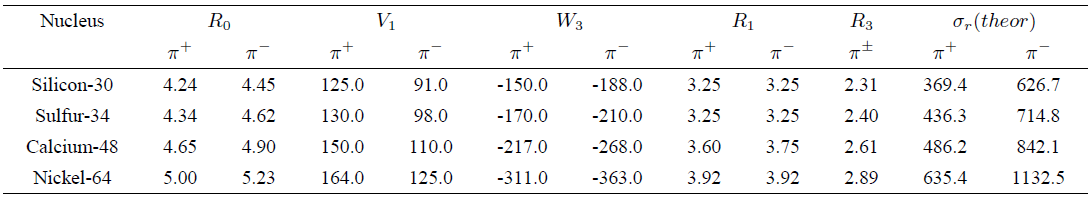From the cases considered herein, the scaling relations are expressed as:

For both charged pions:

R33=0.39A+0.373(fm3)

For positive pions:

R03=1.39A+34.3(fm3)V13=7.27×104A-23.1×104(MeV)3W3=-4.91A+2.54(MeV)

For negative pions:

R03=1.53A+38.3(fm3)V13=3.06×104A-15.6×104(MeV)3W3=-5.48A-22.1(MeV)

For these changed parameters, one may notice the following:

The parameter 𝑅 3 shows a significant increase as 𝐴 increases, which is clearly depicted in Fig. 5. This is in harmony with the noticeable increase of 𝜎 𝑟 with 𝐴, which is attributed to the efficiency of absorption.

The parameter 𝑊 3 shows almost a similar behavior as 𝑅 3 which is again in harmony with the increase of 𝜎 𝑟 with 𝐴, but with higher values for 𝜋 − compared to 𝜋 + . This is more pronounced for nuclei with neutron excess, as shown in Fig. 8, because 𝜋 − favors interaction with neutrons.

The values of the parameter 𝑅 0 , in both 𝜋 − and 𝜋 + scatterings, are close for 𝑁=𝑍 nuclei, but are still higher for 𝜋 − as illustrated in Fig. 6. This is due to the attraction of 𝜋 − with neutrons that are usually pushed closer to the surface of the nucleus. As such larger difference in 𝑅 0 -values appear for nuclei with higher neutron excess.

Since 𝜋 + faces less attraction with neutrons, the strength of the repulsive part, 𝑉 1 , is greater for 𝜋 + , especially for nuclei with neutron excess. As such, and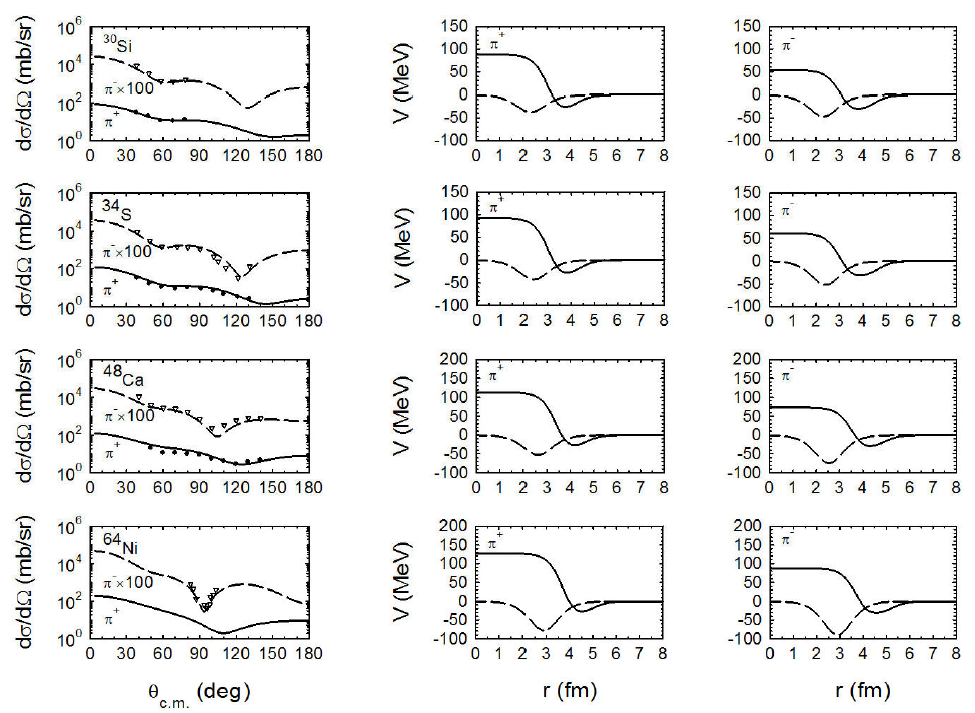Figure 3 In the left side, the calculated differential cross sections, drawn as solid and dashed lines for positive and negative pions, respectively, compared to the experimental data, represented by solid circles and empty triangles [29-31,33], as a function of center of mass angle µc:m: for 50 MeV incident charged pions scattered off the four isotopes 30Si, 34S, 48Ca and 64Ni. The calculations are made by using the scaled potentials, real and imaginary parts, drawn as solid and dashed lines in the middle and right sides of the figure for positive and negative pions, respectively.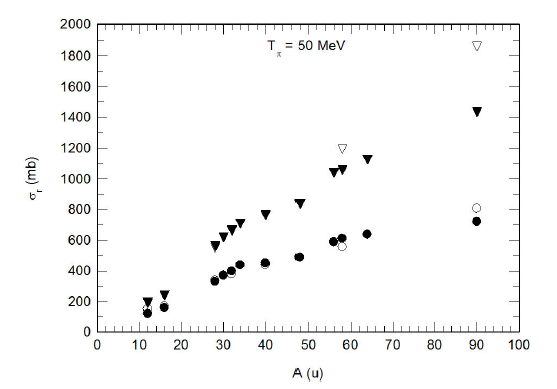Figure 4 The calculated reaction cross sections, drawn as solid circles and solid triangles, are compared to the experimental ones, drawn as empty circles and empty triangles, for positive and negative incident pions, respectively. Empty circles and empty triangles, which are not clearly displaced in the figure, are overlapping with solid circles and solid triangles, respectively.Figure 5 The cube of R3-values versus the atomic mass of the target nucleus in atomic mass units, A(u). The solid line is just to guide the eye.

contrary to 𝑅 0 3 −𝐴 and 𝑊 3 −𝐴 lines, one notices that the 𝑉 1 −𝐴 line for 𝜋 + is higher than the 𝑉 1 −𝐴 line for 𝜋 − as represented in Fig. 7.

With all these sensible physical interpretations, it is also worthwhile to mention that the scaling procedure has its theoretical roots in the energy density functional theory . So it combines the solid theoretical background and the derivedFigure 6 The cube of R0-values versus the atomic mass of the target nucleus in atomic mass units, A(u). The solid and dashed lines, for positive and negative pions, respectively, are just to guide the eye.Figure 7 The cube of V1-values versus the atomic mass of the target nucleus in atomic mass units, A(u). The solid and dashed lines, for positive and negative pions, respectively, are just to guide the eye.

relations based on explaining experimental points. Both are trademarks for a promising suggested scaling method that expected to contribute to advances in nuclear physics.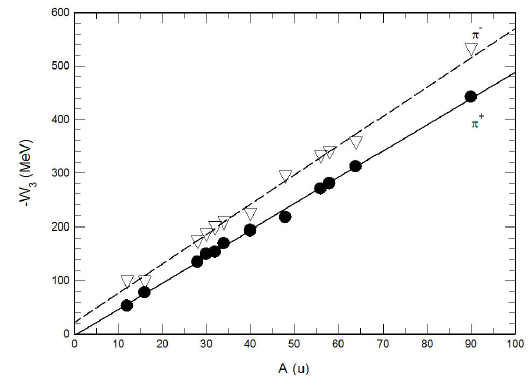Figure 8 TheW3-values versus the atomic mass of the target nucleus in atomic mass units, A(u). The solid and dashed lines, for positive and negative pions, respectively, are just to guide the eye.

4. Conclusions

This study establishes the strength of using the scaling method in obtaining low-energy pion-nucleus reliable potentials. The method is first tested for the two 𝜋 ± − 12 C, 16 O systems with available phase shift analyses. The agreements between the scaled potentials and the ones obtained by inversion are exceptional, and both of them have provided a nice fit to the low-energy 𝜋 ± − 12 C, 16 O elastic scattering data. Hence the use of the scaling procedure has been extended to cover other few pion-nucleus scattering cases with measured angular distributions but no available phase shifts. In addition to 𝜋 ± − 12 C and 𝜋 ± − 16 O, cases, successful results have also been achieved in analyzing the data for 50 MeV charged pions scattered elastically from 28 Si, 32 S, 40 Ca, 56 Fe, 58 Ni, and 90 Zr and the isotopes 30 Si, 34 S, 48 Ca and 64 Ni. The scaled potentials, for the above mentioned nuclear cases, have shown positive simultaneous results summarized in describing differential and integral cross sections and, also, in matching the inversion potentials where possible. Such a remarkable success forms a strong motivation to use the scaling method to comprehensively analyze low-energy pion-nucleus elastic scattering data. With no doubt, and for the first time, these results confirm the scaling method as a strongly nominated alternative / complementary method to the inverse scattering method.

Acknowledgments

The authors are very pleased to acknowledge the encouragement and financial support of the Deanship of Scientific Research at Taif University for carrying out this investigation

REFERENCES

1. J.P. Albanese et al., Nucl. Phys. A 350 (1980) 301-331. [ Links ]

2. J.P. Stroot, Proceedings of LAMPF Summer School on Experiments in Pion Nucleus Physics, (Los Alamos, New Mexico, USA) (1973) 1. [ Links ]

3. T.-S.H. Lee, R.P. Redwine, Annual Review of Nuclear and Particle Science 52 (2002) 23-63. [ Links ]

4. R.M. Al-Shawaf and Z.F. Shehadeh, Int. J. Phys. Sci. 11 (2016) 85-95. [ Links ]

5. E. Friedman, Acta Physica Polonica B 24 (1993) 1673-1684. [ Links ]

6. Z.F. Shehadeh, Journal of Modern Physics 5 (2014) 341-352. [ Links ]

7. G.R. Satchler, Nucl. Phys. A 540 (1992) 533-576. [ Links ]

8. Z.F. Shehadeh, “Non-relativistic nucleus-nucleus and relativistic pion-nucleus interactions, Ph. D. Thesis Southern Illinois University at Carbondale, (1995). [ Links ]

9. D.A. Bromley, J.A. Kuehner, E. Almquist, Phys. Rev. 123 (1961) 878-893. [ Links ]

10. P. Manngard, M. Brenner, M.M. Alam, I. Reichstein and F.B. Malik, Nucl. Phys. A 504 (1989) 130-142. [ Links ]

11. Q. Haider and F.B. Malik, J. Phys. G: Nucl. Phys. 7 (1981) 1661-1669. [ Links ]

12. F.B. Malik and I. Reichstein, Proceedings of In 1st International Conference on Clustering Phenomena in Atoms and Nuclei (Heidelberg, Germany) (1992) 126. [ Links ]

13. A.V. Pozdnyakov, I. Reichstein, Z.F. Shehadeh and F.B. Malik, Condensed Matter Theories 10 (1995) 365-380. [ Links ]

14. Z.F. Shehadeh and F.B. Malik, Bull. Am. Phys. Soc. 36 (1991) 2116. [ Links ]

15. M.S. Sabra, Z.F. Shehadeh and F.B. Malik, Eur. Phys. J.A 27 (2006) 167-181. [ Links ]

16. Z.F. Shehadeh, Journal of Modern Physics 5 (2014) 1652-1661. [ Links ]

17. Z.F. Shehadeh and R.M. El-Shawaf, Rev. Mex. Fis. 62 (2016) 475-483. [ Links ]

18. R.A. Eisenstein and G.A. Miller, Comp. Phys. Commun. 8 (1974) 130-140. [ Links ]

19. Z.F. Shehadeh. J. Phys. 39 (2015) 199-207. [ Links ]

20. W.R. Gibbs, Computations in Modern Physics, (World Scientific, New York, 2006). [ Links ]

21. H.M. Pilkuhn, Relativistic Particle Physics, (Springer-Verlag, New York, 1979). [ Links ]

22. G. Eder, Nuclear Forces: Introduction to Theoretical Nuclear Physics, (The M.I.T Press, Cambridge, Massachusetts, 1968). [ Links ]

23. Z. F. Shehadeh and R. M., Al-Shawaf, A Comprehensive Analysis of Elastic Scattering Data. The Fifth Saudi International Meeting on Frontiers of Physics, 16-18 February 2016 (SIMFP 2016), Jazan University, Gizan, Saudi Arabia. [ Links ]

24. O. Dumbrajs, J. FrÖhlich, U. Klein and H.G. Schlaile, Phys. Rev. C 29 (1984) 581-591. [ Links ]

25. J. FrÖhlich, H.G, Schlaile, L. Streit and H.Z. Zingl, Z. Phys. A-Atoms and Nuclei 302 (1981) 89-94. [ Links ]

26. Z.F. Shehadeh, M.M. Alam and F. B. Malik, Phys. Rev. C 59 (1999) 826-831. [ Links ]

27. B.M. Preedom et al., Phys. Rev. C 23 (1981) 1134-1140. [ Links ]

28. S. A. Dytman et al., Phys. Rev. C 19 (1979) 971-986. [ Links ]

29. K.K. Seth et al., Phys. Rev. C 41 (1990) 2800-2808. [ Links ]

30. R.J. Sobie et al., Phys. Rev. C 30 (1984) 1612-1621. [ Links ]

31. C.S. Mishra, Phys. Rev. C 38 (1988) 1316-1321. [ Links ]

32. T. Ericson and W. Weise, Pions and Nuclei, (Clarendon Press, Oxford, 1988). [ Links ]

33. U. Wienands et al., Phys. Rev. C 35 (1987) 708-717. [ Links ]

34. M.A. Moinester et al., Phys. Rev. C 18 (1978) 2678-2682. [ Links ]

35. A. Sanders, Phys. Rev. C 53 (1996) 1745-1752. [ Links ]

36. D.J. Malbrough et al., Phys. Rev. C 17 (1978) 1395-1401. [ Links ]

37. A.A. Ebrahim and S.A.E. Khallaf, Acta Physica Polonica B 36 (2005) 2071-2085. [ Links ]

38. K. Stricker, H. McManus and J.A. Carr, Phys. Rev. C 19 (1979) 929-947. [ Links ]

Received: January 09, 2017; Accepted: February 23, 2017This is an open-access article distributed under the terms of the Creative Commons Attribution License